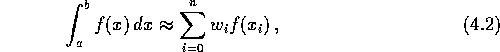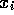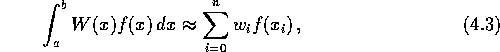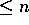Next: Optimization Up: Numerical Methods Previous: Introduction

# Integration

The integral in equation (4.1), including its first and second derivatives, has to be computed at least once in each iteration, usually several times, and therefore the choice of method and accuracy has to be made with care. There are several numerical integration method, e.g. Trapezoid, Simpson, Romberg, and Gaussian quadrature.I will take a closer look at the latter one.

The Gaussian quadrature is a generalization of the the Newton-Cotes formula,whereare the abscissas andthe weights. Newton-Cotes is a polynomial interpolation, where the abscissas are equally spaced in the interval (a,b). Trapezoid and Simpson are both a variation of this interpolation. The idea of the Gaussian quadrature is that the abscissas are no longer restricted to be equally spaced, and that they can be chosen to give higher accuracy. Another attracting feature is that the weights and abscissas can be arranged to make the integral exact when the integrand is a product of a function f(x) and and a weight function W(x). The weight function can be chosen to remove singularities. The rewritten Newton-Cotes formula is thenand is exact if f(x) is a polynomial of degree. The abscissas and the weights are determined by a set of polynomials that are orthogonal over the weight function W(x). For quite a few ``classical'' orthogonal polynomials for a given weight function, abscissas and weights are either tabulated or can be found by given algorithms Press.

In this treatise, Gaussian quadrature with weight functionhas been applied, and has proven to be very efficient.It is highly superior to other methods like the Trapezoid and the Simpson method, both when it comes to speed and accuracy. To achieve satisfactory accuracy in calculating equation (4.1) to, say, seven significant figures, 10 abscissas are needed, thus requiring the calculations of the integrand at only 10 points in time. The argument of Gia applies here: If the number of abscissas used in the Gaussian quadrature exceeds by one the number N in the truncated expansion in equation (3.21), the error introduced by the Gaussian quadrature is totally negligible compared with the error in the truncated expansion itself.

The abscissas and weights used can be easily deduced from the respective abscissas and weights for the Gauss-Legendre quadrature;where GL denote Gauss-Legendre [page 276]NM.

The Gaussian quadrature method with the weight function chosen here seems to be comparable to the one used by Gia, and greatly superior to the integration methods used otherwise in connection with the AVP.Next: Optimization Up: Numerical Methods Previous: Introduction

Trond Hjorteland
Mon Jul 5 02:59:28 MET DST 1999